Courses

# Test: Past 28 Years Questions: Alternating Current

## 36 Questions MCQ Test Physics Class 12 | Test: Past 28 Years Questions: Alternating Current

Description
This mock test of Test: Past 28 Years Questions: Alternating Current for NEET helps you for every NEET entrance exam. This contains 36 Multiple Choice Questions for NEET Test: Past 28 Years Questions: Alternating Current (mcq) to study with solutions a complete question bank. The solved questions answers in this Test: Past 28 Years Questions: Alternating Current quiz give you a good mix of easy questions and tough questions. NEET students definitely take this Test: Past 28 Years Questions: Alternating Current exercise for a better result in the exam. You can find other Test: Past 28 Years Questions: Alternating Current extra questions, long questions & short questions for NEET on EduRev as well by searching above.
QUESTION: 1

### Eddy currents are produced when 

Solution:

Eddy currents are produced when a metal is
kept in a varying magnetic field.

QUESTION: 2

### Energy in a current carrying coil is stored in theform of   

Solution:

Energy stored in a coil =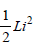where, L is the self-inductance and i the
current flowing through the inductor. Thus,
energy is stored in the magnetic field of the
coil.

QUESTION: 3

### In a region of uniform magnetic inductionB = 10–2 tesla, a circular coil of radius 30 cm andresistance π2 ohm is rotated about an axis whichis perpendicular to the direction of B and whichforms a diameter of the coil. If the coil rotates at 200 rpm the amplitude of the alternating currentinduced in the coil is 

Solution: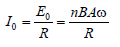Given, n = 1, B = 10–2 T,
A = π(0.3)2m2, R = π2
f= (200/60) and ω = 2π(200/60)
Substituting these values and solving, we
get
I0 = 6 × 10–3 A = 6mA

QUESTION: 4

In the circuit of Fig, the bulb will become suddenly bright if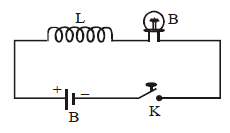Solution:

When contact is broken, current in the circuit decreases. To oppose the inductor releases current and emf is induced. This induced emf is having high value. Hence, The bulb will become suddenly bright.

The correct option is C.

QUESTION: 5

In which of the following, emission of electronsdoes not take place 

Solution:

Thermionic emission : When a metal is
heated to a high temperature, the free
electrons gain kinetic energy and escape
from the surface of the metal.

Secondary emission : When an electron
strikes the surface of a metallic plate, it emits
other electrons from the surface.

Photoelectric emission : Emission of
electrons from the metal surface on irradiation
with radiation of suitable frequency.

X-rays emission : High speed electrons
(emitted from a filament) striking a target
(a copper block) to emit X-rays.

QUESTION: 6

The time constant of C–R circuit is 

Solution:

The time constant for resonance circuit,
C = CR
Growth of charge in a circuit containing
capacitance and resistance is given by the
formula,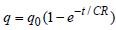CR is known as time constant in this formula.

QUESTION: 7

In an a.c. circuit, the r.m.s. value of current, irms is related to the peak current, i0 by the relation

Solution: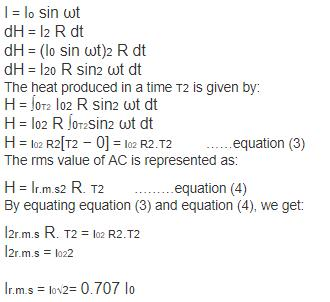QUESTION: 8

An LCR series circuit is connected to a source of alternating current. At resonance, the applied voltage and the current flowing through the circuit will have a phase difference of 

Solution:

At resonance,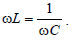The circuit
behaves as if it contains R only. So, phase
difference = 0
At resonance, impedance is minimum Zmin =
R and current is maximum, given by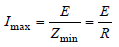It is interesting to note that before resonance
the current leads the applied emf, at
resonance it is in phase, and after resonance
it lags behind the emf. LCR series circuit is
also called as acceptor circuit and parallel
LCR circuit is called rejector circuit.

QUESTION: 9

In an experiment, 200 V A.C. is applied at theends of an LCR circuit. The circuit consists ofan inductive reactance (XL ) = 50 Ω, capacitivereactance (XC ) = 50 Ω and ohmic resistance (R)= 10 Ω. The impedance of the circuit is

Solution:

Given : Supply voltage (Vac) = 200 V
Inductive reactance (XL) = 50 Ω
Capacitive reactance (XC) = 50 Ω
Ohmic resistance (R) = 10 Ω.
We know that impedance of the LCR circuit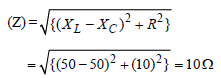QUESTION: 10

In an A.C. circuit with voltage V and current I the power dissipated is 

Solution:

Power dissipated = Erms. Irms = (Erms) (Irms)
cos θ
Hence, power dissipated depends upon
phase difference.

QUESTION: 11

The primary winding of a transformer has 500 turns whereas its secondary has 5000 turns. Theprimary is connected to an A.C. supply of 20 V,50 Hz. The secondary will have an output of        

Solution:

The transformer converts A.C. high voltage
into A.C. low voltage, but it does not cause
any change in frequency. The formula for
voltage is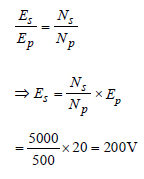Thus, output has voltage 200 V and
frequency 50 Hz.

QUESTION: 12

A step-up transformer operates on a 230 V lineand supplies a load of 2 ampere. The ratio of theprimary and secondary windings is 1 : 25. Thecurrent in the primary is 

Solution: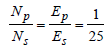∴ Es = 25 Ep
But EsIs = EpIp
25Ep × 2 = Ep × Ip ⇒ Ip = 5 0 A

QUESTION: 13

An inductance L having a resistance R is connected to an alternating source of angular frequency ω. The quality factor Q of the
inductance is 

Solution:

Quality factor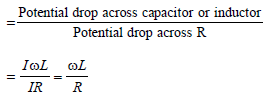QUESTION: 14

A capacitor has capacity C and reactance X. Ifcapacitance and frequency become double, thenreactance will be 

Solution:

Capacitive reactance,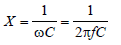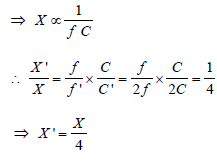QUESTION: 15

In a series resonant circuit, having L, C and R as its elements, the resonant current is i. The power dissipated in the circuit at resonance is 

Solution:

At resonance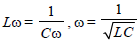Current through circuit i =E/R
Power dissipated at Resonance = i2R

QUESTION: 16

A coil of 40 henry inductance is connected inseries with a resistance of 8 ohm and thecombination is joined to the terminals of a 2 voltbattery. The time constant of the circuit is    

Solution:

Time constant is L/R
Given, L = 40H & R = 8Ω
∴ τ = 40/8 = 5 sec

QUESTION: 17

In a circuit, L, C and R are connected in series with an alternating voltage source of frequency f. The current leads the voltage by 45°. The value of C is 

Solution:

QUESTION: 18

A coil of inductive reactance 31 Ω has aresistance of 8 Ω. It is placed in series with acondenser of capacitative reactance 25Ω. Thecombination is connected to an a.c. source of110 volt. The power factor of the circuit is

Solution: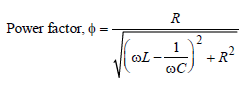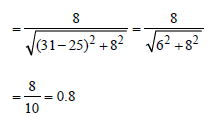QUESTION: 19

The core of a transformer is laminated because        

Solution:

When there is change of flux in the core of a
transformer due to change in current around
it, eddy current is produced. The direction
of this current is opposite to the current
which produces it, so it will reduce the main
current. We laminate the core so that flux is
reduced resulting in the reduced production
of eddy current.

QUESTION: 20

A transistor-oscillator using a resonant circuitwith an inductor L (of negligible resistance) anda capacitor C in series produce oscillations offrequency f. If L is doubled and C is changed to4C, the frequency will be 

Solution:

We know that frequency of electrical
oscillation in L.C. circuit is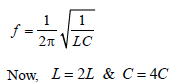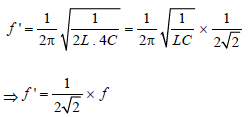QUESTION: 21

The primary and secondary coil of a transformerhave 50 and 1500 turns respectively. If themagnetic flux φ linked with the primary coil isgiven by φ= φ0 + 4t, where φ is in webers, t is timein seconds and φ0 is a constant, the outputvoltage across the secondary coil is 

Solution: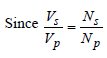Where
Ns = No. of turns across primary coil = 50
Np = No. of turns across secondary coil
= 1500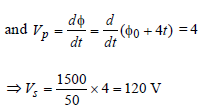QUESTION: 22

A transformer is used to light a 100 W and 110 Vlamp from a 220 V mains. If the main current is 0.5amp, the efficiency of the transformer isapproximately 

Solution:

Efficiency of the transformer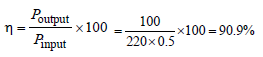QUESTION: 23

What is the value of inductance L for which the current is maximum in a series LCR circuit with C = 10 μF and ω = 1000s -1 ? 

Solution:

Condition for which the current is maximum
in a series LCR circuit is,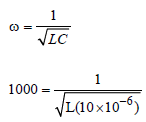= L = 100 mH

QUESTION: 24

In an a.c circuit the e.m.f. (e) and the current (i) at any instant are given respectively by
e = E0 sin ωt         
i = I0 sin (ωt –φ)
The average power in the circuit over one cycle of a.c. is

Solution:

The average power in the circuit over one
cycle of a.c. is given by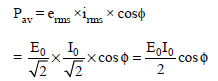QUESTION: 25

Power dissipated in an LCR series circuit connected to an a.c source of emf εε is 

Solution:

Power dissipated in series LCR;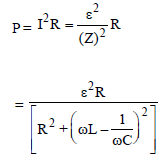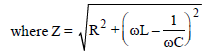is called the impedance of the circuit.

QUESTION: 26

In the given circuit the reading of voltmeter V1 and V2 are 300 volts each. The reading of the voltmeter V3 and ammeter A are
respectively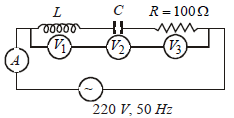Solution: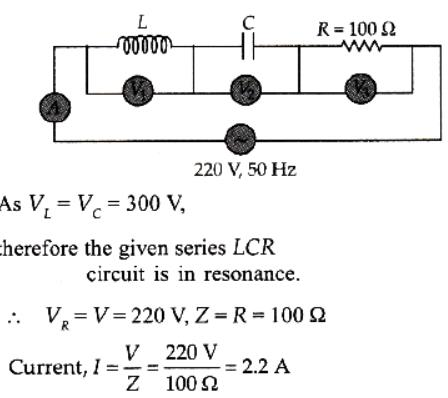Hence, the reading of the voltmeter V3 is 220 V and the reading of ammeter A is 2.2 A.

QUESTION: 27

A 220 volts input is supplied to a transformer.The output circuit draws a current of 2.0 ampereat 440 volts. If the efficiency of the transformeris 80%, the current drawn by the primarywindings of the transformer is 

Solution: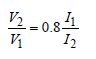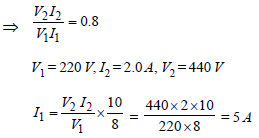QUESTION: 28

An ac voltage is applied to a resistance R and aninductor L in series. If R and the inductivereactance are both equal to 3Ω, the phasedifference between the applied voltage and thecurrent in the circuit is 

Solution:

The phase difference φ is given by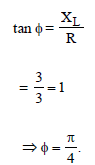QUESTION: 29

In an ac circuit an alternating voltage e = 200 √2 sin 100 t volts is connected to a capacitorof capacity 1μF. The r.m.s. value of the currentin the circuit is 

Solution:

given e=200√2​sin100t
ω=100 rad​/sec
Erms​= 200√2​​/√2​
C=1×10−6
Z=Xc​=1/ωC​=1/100×10−6=104Ω
I=Erms​​/Z=(200√2​​​/√2​)/104 =2×10−2A=20mA

QUESTION: 30

The r.m.s. value of potential difference V shown in the figure is [2011M]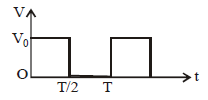Solution:

Voltage is given as    V=Vo​   for   0 ≥ t ≤ T/2
=0      for   T/2 ≥ t ≤T
Rms voltage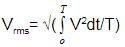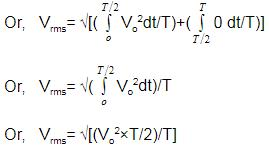⟹ Vrms​​=Vo​/√2​

QUESTION: 31

A coil has resistance 30 ohm and inductive reactance 20 ohm at 50 Hz frequency. If an ac source, of 200 volt, 100 Hz, is connected across the coil, the current in the coil will be [2011M]

Solution:

If ω = 50 × 2π then ωL = 20Ω
If ω' = 100 × 2π then ω'L = 40Ω
Current flowing in the coil is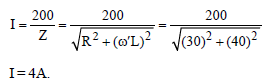QUESTION: 32

In an electrical circuit R, L, C and an a.c. voltage source are all connected in series. When L is removed from the circuit, the phase difference between the voltage the current in the circuit is π/3. If instead, C is removed from the circuit, the phase difference is again π/3. The power factor of the circuit is : 

Solution: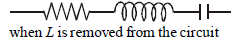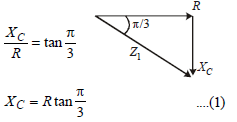when C is remove from the circuit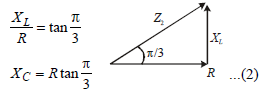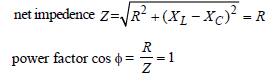QUESTION: 33

The current (I) in the inductance is varying with time according to the plot shown in figure.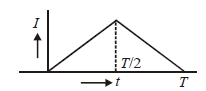Which one of the following is the correct variation of voltage with time in the coil?

Solution: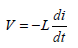Here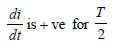time and di/dt  is -ve for next T/2 time so.

QUESTION: 34

The instantaneous values of alternating current and voltages in a circuit are given as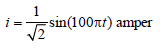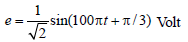The average power in Watts consumed in the circuit is : [2012M]

Solution:

The average power in the circuit where cos
φ = power factory

< P > = Vrms × Irms cos φ
φ = π/3 = phase difference =180/3 =  60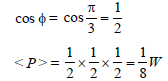QUESTION: 35

A coil of self-inductance L is connected in serieswith a bulb B and an AC source. Brightness ofthe bulb decreases when

[NEET 2013]

Solution:

By inserting iron rod in the coil,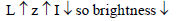QUESTION: 36

The primary of a transformer when connected toa dc battery of 10 volt draws a current of 1 mA.The number of turns of the primary andsecondary windings are 50 and 100 respectively.The voltage in the secondary and the currentdrawn by the circuit in the secondary arerespectively [NEET Kar. 2013]

Solution:

A transformer is essentially an AC device.
DC source so no mutual induction between
coils
⇒ E2 = 0 and I2 = 0